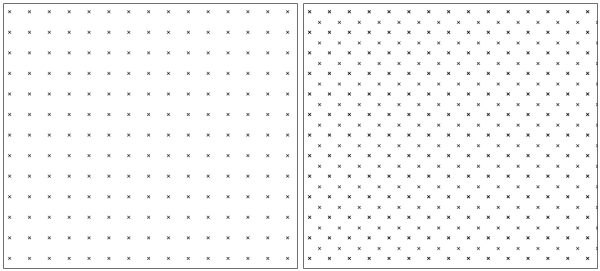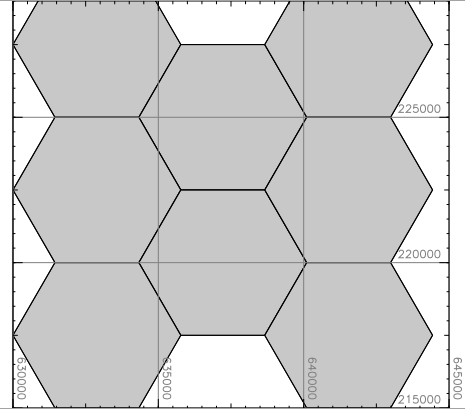## NAME

v.mkgrid - Creates a vector map of a user-defined grid.

## KEYWORDS

vector, geometry, grid, point pattern, hexagon

## SYNOPSIS

v.mkgrid
v.mkgrid --help
v.mkgrid [-had] map=name [grid=rows,columns] [position=string] [coordinates=east,north] [box=width,height] [angle=float] [breaks=integer] [type=string] [--overwrite] [--help] [--verbose] [--quiet] [--ui]

### Flags:

-h
Create hexagons (default: rectangles)
-a
Allow asymmetric hexagons
-d
EXPERIMENTAL: Add diagonals to rectangular lines
Applies only to lines for rectangles
--overwrite
Allow output files to overwrite existing files
--help
Print usage summary
--verbose
Verbose module output
--quiet
Quiet module output
--ui
Force launching GUI dialog

### Parameters:

map=name [required]
Name for output vector map
grid=rows,columns
Number of rows and columns in grid
position=string
Where to place the grid
Options: region, coor
Default: region
region: current region
coor: use 'coor' and 'box' options
coordinates=east,north
Lower left easting and northing coordinates of map
box=width,height
Width and height of boxes in grid
angle=float
Angle of rotation (in degrees counter-clockwise)
Default: 0
breaks=integer
Number of vertex points per grid cell
Options: 0-60
Default: 0
type=string
Output feature type
Options: point, line, area
Default: area

## DESCRIPTION

v.mkgrid creates a vector map representation of a regular coordinate grid. Point, line, and area vector grids can be created.

## NOTES

Grid points created with the type=point option will be placed at the center of each grid cell, like centroids with the default type=area option.

Grid lines created with the type=line option will be identical to the edges of each grid cell, like boundaries with the default type=area option.

The resultant grid can be rotated around the origin (center of the grid) with the angle option.

Optionally hexagons can be created with the -h flag. Hexagons are by default symmetric. Asymmetric hexagons can be allowed with the -a flag.

This module is NOT to be used to generate a vector map of USGS quadrangles, because USGS quads are not exact rectangles.

## EXAMPLES

### Creating a global grid in a latitude-longitude

To be run in a latitude-longitude location (WGS84)
```# set the region:
g.region n=90 s=-90 w=-180 e=180 res=10 -p
projection: 3 (Latitude-Longitude)
zone:       0
datum:      wgs84
ellipsoid:  wgs84
north:      90N
south:      90S
west:       180W
east:       180E
nsres:      10
ewres:      10
rows:       18
cols:       36
cells:      648

# create 10 degree size grid:
v.mkgrid map=grid_10deg

# create 20 degree size grid:
v.mkgrid map=grid_20deg box=20,20
```

### Creating a grid in a metric projection

Creating a 4x3 grid, cells 20km a side, with lower left corner at 2716500,6447000:
```v.mkgrid map=coro_grid grid=4,3 position=coor coordinates=2716500,6447000 box=20000,20000
```

### Creating a positioned grid in a latitude-longitude

Creating a 10x12 lat/lon grid, cells 2 arc-min a side, with lower left corner at 167deg 52min east, 47deg 6min south. For use with e.g. QGIS you can then pull this grid into a projected location with v.proj before exporting as a Shapefile with v.out.ogr (within GRASS GIS you could just use d.grid -w from the projected location for the same effect):
```v.mkgrid map=p2min_grid grid=10,12 position=coor coordinates=167:52E,47:06S box=0:02,0:02
```

### Creating a simple point pattern

North Carolina sample dataset example, creating a 1km spaced point grid based on the current region extent defined by the "elevation" map:
```g.region raster=elevation res=1000 -pa
v.mkgrid type=point map=pointpattern
```

### Creating a regular point pattern

North Carolina sample dataset example, creating a regular spaced point grid based on the current region extent defined by the "elevation" map, using a two-step approach:
```# create first set of points, covering extent of "elevation" raster map
g.region raster=elevation res=1000 -pa
v.mkgrid type=point map=pointpattern1

# shift grid by half point distance (map units)
g.region n=n+500 w=w+500 e=e+500 s=s+500 -p

# create second set of points
v.mkgrid type=point map=pointpattern2

# merge into final point pattern
v.patch input=pointpattern1,pointpattern2 output=pointpattern3
```Different point patterns for sampling design

### Creating hexagons in a metric projection

North Carolina sample dataset example, creating regular hexagons based on the current region extent defined by the "elevation" map and raster resolution for the hexagon size:
```g.region raster=elevation res=5000 -pa
v.mkgrid map=hexagons -h

d.grid 5000
```Hexagon map

### Using hexagons for point density

To compute point density in a hexagonal grid for the vector map points_of_interest in the basic North Carolina sample dataset, the vector map itself is used to set extent of the computational region. The resolution is based on the desired size of hexagons.
```g.region vector=points_of_interest res=2000 -pa
```
The hexagonal grid is created as a vector map based on the previously selected extent and size of the grid.
```v.mkgrid map=hexagons -h
```
The following counts the number of points per hexagon using the v.vect.stats module.
```v.vect.stats points=points_of_interest areas=hexagons count_column=count
```
User should note that some of the points may be outside the grid since the hexagons cannot cover all the area around the edges (the computational region extent needs to be enlarged if all points should be considered). The last command sets the vector map color table to viridis based on the count column.
```v.colors map=hexagons use=attr column=count color=viridis
```Point density in a hexagonal grid

d.grid, v.in.region, v.patch, v.vect.stats

## AUTHORS

Michael Higgins, U.S.Army Construction Engineering Research Laboratory

Update for new vectors Radim Blazek 10/2004

## SOURCE CODE

Available at: v.mkgrid source code (history)

© 2003-2021 GRASS Development Team, GRASS GIS 8.0.dev Reference Manual